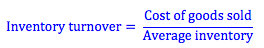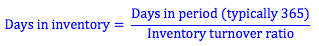Explore BrainMass

# Inventory Turnover

The inventory turnover ratio is calculated by dividing the cost of goods sold by the average inventory. Because the cost of goods sold is calculated based on the value of the inventory sold, and does not include a measure of profit that total sales does, it gives us the correct value for the amount of inventory sold during a period.We can also use the inventory turnover ratio to calculate the days in inventory, or the 'shelf life' as it is called by retail firms. The days in inventory tells us how long it takes a firm to produce and sell a unit of inventory. We calculate the days in inventory by dividing the days in the accounting period (365 in a year) by the inventory turnover ratio.The inventory turnover ratio is greatly affected by the production technology used by the firm. A manufacturing firm producing large-scale goods such as planes will have a much higher days in inventory (and therefore lower inventory turnover ratio) than a manufacturing firm producing widgets. Similarly, a retail firm that buys, rather than produces, its inventory will have a lower days in inventory. A business that sells perishable goods such as meat, fish or produce will have an even lower days in inventory due to the fact that the products have a short shelf life.

One thing we must be careful of when looking at a firm’s inventory turnover is the valuation method used to calculate inventory.  Using LIFO, FIFO or a weighted-average will give different valuations of the same inventory, and therefore have a significant affect on the calculated value for inventory turnover.